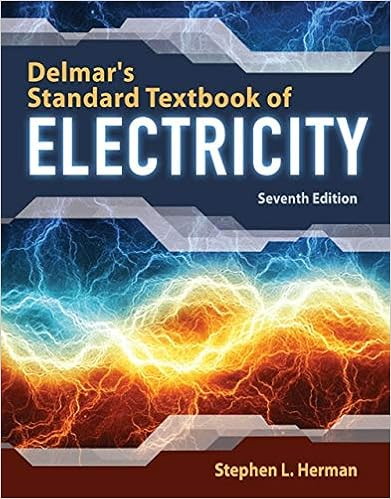# lab report Moments of Inertia - Lab Report 10 Moments of...

• Lab Report
• 8
• 96% (27) 26 out of 27 people found this document helpful

This preview shows page 1 - 4 out of 8 pages.

##### We have textbook solutions for you!
The document you are viewing contains questions related to this textbook.The document you are viewing contains questions related to this textbook.
Chapter 33 / Exercise 30
Delmar's Standard Textbook of Electricity
HermanExpert Verified
Lab Report 10: Moments of Inertia Abstract In this experiment, we have learned how to dynamically measure the moment of inertia of a rotating system and to compare this to a predicted value. The moment of inertia can be viewed as the rotating analog of mass. In rotational dynamics, newton’s second Law (F=ma) becomes t=Ia, where t is the net applied torque, I is the moment of inertia of the body and a is the angular
##### We have textbook solutions for you!
The document you are viewing contains questions related to this textbook.The document you are viewing contains questions related to this textbook.
Chapter 33 / Exercise 30
Delmar's Standard Textbook of Electricity
HermanExpert Verified
acceleration. In this lab, we can find the moment of inertia by applying a known torque to a rigid body, measuring the angular acceleration and using the relationship t=Ia. Throughout the experiment, there are several sources of uncertainty. First, the human reaction delay in using the stopwatch when using the stopwatch to time the fall in order to calculate the acceleration. Also it was the difficulty determining whether the rotational apparatus was working in a constant speed or not without any measured tool. Another likely source is friction in some form, as it would increase the amount of time taken for the counterweight to reach the ground. Two potential sources of increased friction observed at various points during the experiment were the string becoming trapped between the rotating and non-rotating parts of the table axle. Results presentation (1) Final result
Hanging mass(g) Calculated Friction(N) Calculated Tension (N) Calculated Torque (N-m) Calculated Acceleration (m/s^2) Calculated angular Acceleration(rads/s^2) Moment of Inertia (Kg- m^2) Part1(1) 20+frction(mass) 0.049 0.245 0.00475 0.0059 0.231 0.021 Part1(2) 40+frction(mass) 0.049 0.392 0.00874 0.0112 0.498 0.018 Part2(1) 20+frction(mass) 0.196 0.392 0.005 0.0017 0.065 0.195 Part2(2) 40+frction(mass) 0.196 0.588 0.01 0.0028 0.108 0.211 (2) Mass Mass (g, kg) Note Part1 step 2 Weight attached on string 5g = 0.005kg
•••